## Monday, 5 December 2011

### CBSE Class 8 - (Ch11) - Mensuration

Q1. The area of the floor of a rectangular hall of length 40m is 960 m2. Carpets of size 6m x 4m are available. Find how many carpets are required to cover the hall. (Unsolved Exercise 16.1 from RD Sharma)
length = 6m    breadth = 4m  and Area = l x b
$\therefore$ Area of carpet is = 6 x 4 = 24 m2.
Floor area = 960 m2
Number of carpets =

Q2: Find the area of a square the length of whose diagonal is 2.9 meters. (Unsolved Ex 16.1 from RDS)
Answer: Diagonal (d) = 2.9 m. Let x be the length of each side of the square.
Applying Pythagoras theorem,
$x^ + x^ = 2x^ = (2.9)^2$
$\Rightarrow x^2 = \text {area of square} = \frac{(2.9)^2}{2} = \frac{8.41}{2} = 4.205 m^2$

Q3: The area of a square field is 0.5 hectares. Find the length of its diagonal in meters.
Answer: 1 hect = 10000 m2
$\therefore$ 0.5 hec = 5000 m2 $Applying Pythagoras theorem,$ \frac{diagonal^2}{2} = 5000\Rightarrow diagonal = \sqrt{10000} = 100 m$Q4: The diameter of a semi-circular field is 14 meters. What is the cost of fencing the plot at Rs. 10 per meter.Answer: Diameter of field (d) = 14m$\Rightarrow r = 14 \div 2 = 7m \text{Perimeter of semicircle} = (\pi + 2) \times r \Rightarrow = (\frac{22}{7} + 2) \times 7 = 36m $Cost of fencing per meter = Rs 10$\therefore \text{cost of fencing for 36m} = 36 \times 10 = \text{Rs }360$Q5: Find the area of the quadrilateral ABCD as shown. The diagonal AC is 84 units and perpendiculars BE and FD are 21 and 28 units respectively. Answer: The area of a general quadrilateral =$\frac{1}{2}d \times (h_1 + h_2).\$
Here d = 84, h1 = 21 units and h2 = 28 units.
Therefore area = 84 * (21+28)/2 = 2058 square units. (Answer).1.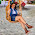thanks..............my papers are going good for you.

2.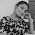it gives us idea of questions. thank u so much!!!!!!

3.very nice...........

4.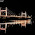ya tnx 2 u and yr ans is so gud.,.,.,

5.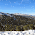6.7.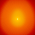thank you for giving me a wonderful platform